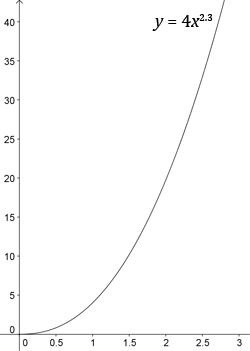# Modelling Curves

Unfortunately when you do experiments it is unusual to find that the relationship you are studying is linear. Linear relationships are nice because you can plot them, draw a line of best fit, and use $y = mx + c$ to find an empirical equation to model the behaviour of the experiment in question.
More commonly you will need to deal with non-linear relationships. In C2 you can model curves of the form $y = kx^n$ and $y = ka^x$. Examples of these forms of graph are shown below: